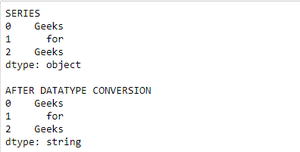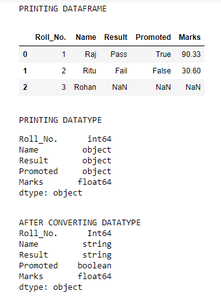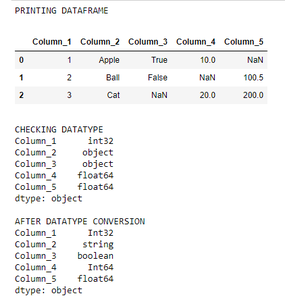# How to Convert to Best Data Types Automatically in Pandas?

Prerequisite: Pandas

In pandas datatype by default are int, float and objects. When we load or create any series or dataframe in pandas, pandas by default assigns the necessary datatype to columns and series.

We will use pandas convert_dtypes() function to convert the default assigned data-types to the best datatype automatically. There is one big benefit of using convert_dtypes()- it supports new type for missing values pd.NA along with NaN. It is supported in pandas 1.1.4 version.

Syntax:

For Series:

`series_name.convert_dtypes()`

For DataFrame:

`dataframe_name.convert_dtypes().dtypes`

The following is the implementation for both series and data frame:

Converting the datatype of a series:

• Import module
• Create a series
• Now use convert_dtypes() function to automatically convert datatype

Example:

## Python3

 `# importing packages ` `import` `pandas as pd ` ` `  `# creating a series ` `s ``=` `pd.Series([``'Geeks'``, ``'for'``, ``'Geeks'``]) ` ` `  `# printing the series ` `print``(``"SERIES"``) ` `print``(s) ` ` `  `print``() ` ` `  `# using convert_dtypes() function ` `print``(``"AFTER DATATYPE CONVERSION"``) ` `print``(s.convert_dtypes()) `

Output:Converting the datatype of a dataframe:

• Import module
• Create data frame
• Check data type
• Convert data type using convert_dtypes().dtypes function

The data type of columns are changed accordingly. But the datatype of dataframe will remain object because it contains multiple columns with each column has a different datatype.

Example:

## Python3

 `import` `pandas as pd ` `import` `numpy as np ` ` `  `# creating a dataframe ` `df ``=` `pd.DataFrame({``"Roll_No."``: ([``1``, ``2``, ``3``]), ` `                   ``"Name"``: [``"Raj"``, ``"Ritu"``, ``"Rohan"``], ` `                   ``"Result"``: [``"Pass"``, ``"Fail"``, np.nan], ` `                   ``"Promoted"``: [``True``, ``False``, np.nan], ` `                   ``"Marks"``: [``90.33``, ``30.6``, np.nan]}) ` ` `  `# printing the dataframe ` `print``(``"PRINTING DATAFRAME"``) ` `display(df) ` ` `  `# checking datatype ` `print``() ` `print``(``"PRINTING DATATYPE"``) ` `print``(df.dtypes) ` ` `  `# converting datatype ` `print``() ` `print``(``"AFTER CONVERTING DATATYPE"``) ` `print``(df.convert_dtypes().dtypes) `

Output:Creating the Data frame through series and specifying datatype :

• Import module
• Create dataframe through series and specify datatype along with it
• Check data type
• Convert using convert_dtypes().dtypes function

Example:

## Python3

 `import` `pandas as pd ` `import` `numpy as np ` ` `  `# Creating the Data frame through series ` `# and specifying datatype along with it ` `df ``=` `pd.DataFrame({``"Column_1"``: pd.Series([``1``, ``2``, ``3``], dtype``=``np.dtype(``"int32"``)), ` `                   ``# Column_1 datatype is int32 ` `                    `  `                   ``"Column_2"``: pd.Series([``"Apple"``, ``"Ball"``, ``"Cat"``],  ` `                                         ``dtype``=``np.dtype(``"object"``)), ` `                   ``# Column_2 datatype is 0 ` `                    `  `                   ``"Column_3"``: pd.Series([``True``, ``False``, np.nan],  ` `                                         ``dtype``=``np.dtype(``"object"``)), ` `                   ``# Column_3 datatype is 0 ` `                    `  `                   ``"Column_4"``: pd.Series([``10``, np.nan, ``20``],  ` `                                         ``dtype``=``np.dtype(``"float"``)), ` `                   ``# Column_4 datatype is float ` `                    `  `                   ``"Column_5"``: pd.Series([np.nan, ``100.5``, ``200``], ` `                                         ``dtype``=``np.dtype(``"float"``))}) ` `                   ``# Column_5 datatype is float ` ` `  `# printing dataframe ` `print``(``"PRINTING DATAFRAME"``) ` `display(df) ` ` `  `# checking datatype ` `print``() ` `print``(``"CHECKING DATATYPE"``) ` `print``(df.dtypes) ` ` `  `# convert datatype ` `print``() ` `print``(``"AFTER DATATYPE CONVERSION"``) ` `print``(df.convert_dtypes().dtypes) `

Output:Whether you're preparing for your first job interview or aiming to upskill in this ever-evolving tech landscape, GeeksforGeeks Courses are your key to success. We provide top-quality content at affordable prices, all geared towards accelerating your growth in a time-bound manner. Join the millions we've already empowered, and we're here to do the same for you. Don't miss out - check it out now!